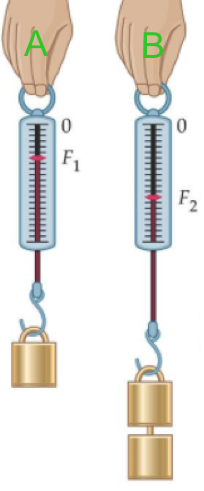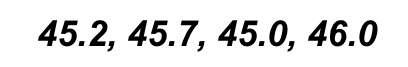Final Precision & Accuracy Retake Form
First Name *
Last Name *
Email *
Use the image below to answer the true or false questions below (Questions 1-6)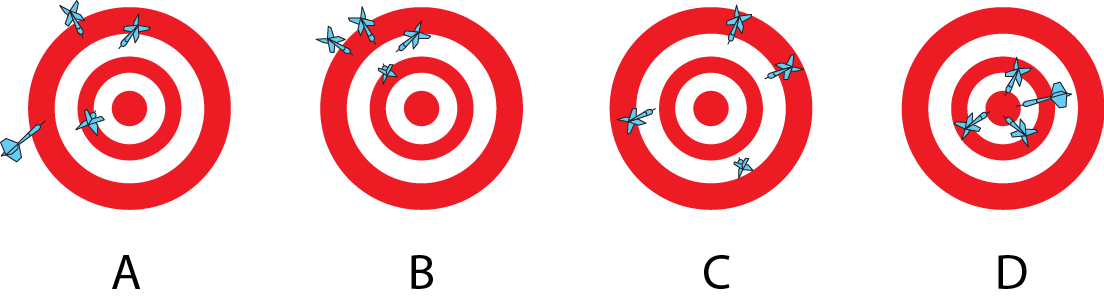1) Target A and B have similar percentage error? *
2) Target A and B have similar percentage uncertainty? *
3) Target A and C have similar accuracy? *
4) Target B and D have similar precision? *
5) Target B has a larger percentage error than target D? *
6) Target B has a larger percentage uncertainty than target C? *
Use the image below to answer questions 7-8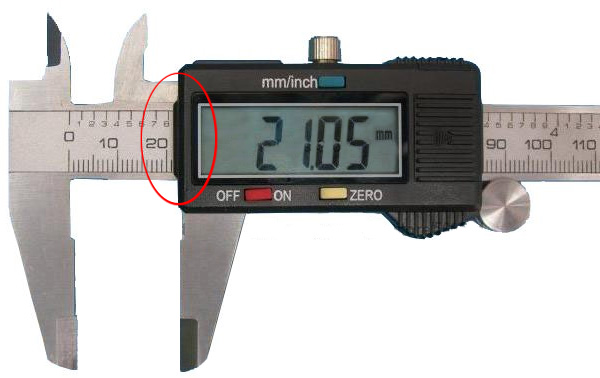7) What is the uncertainty of DIGITAL measurement tool? *
8) What is the percentage uncertainty for the measurement in the picture? (round to the HUNDREDTHS place and do not add the % symbol) *
9) Which of the following has correctly drawn max and min slope lines? *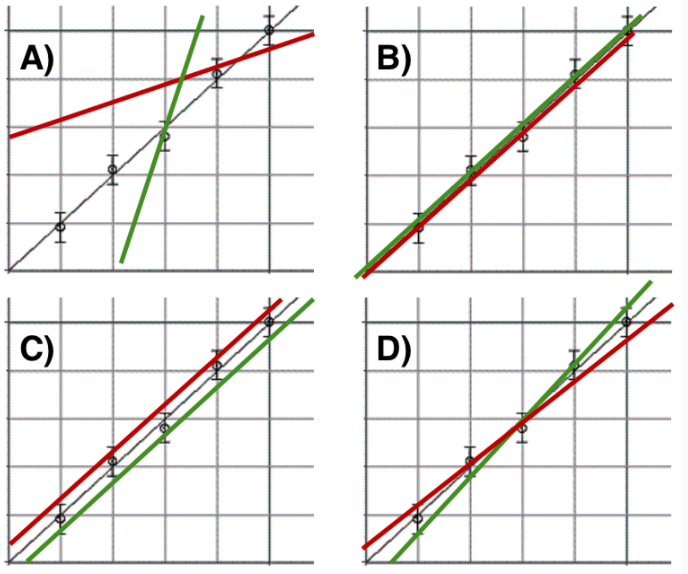10) What is the slope of the best fit line above (Line B)(Round your answer to the HUNDREDTHS place) *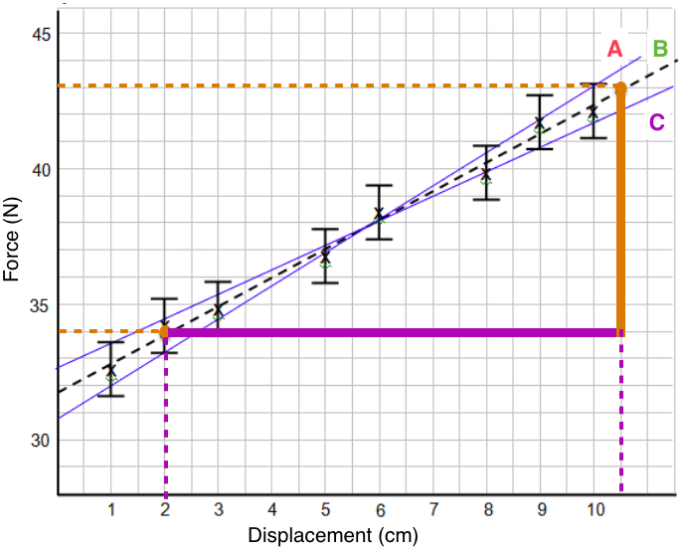11) What are the units for the slope of the best fit line? *
12) What is the uncertainty in the slope? (round to the HUNDRETHS place) *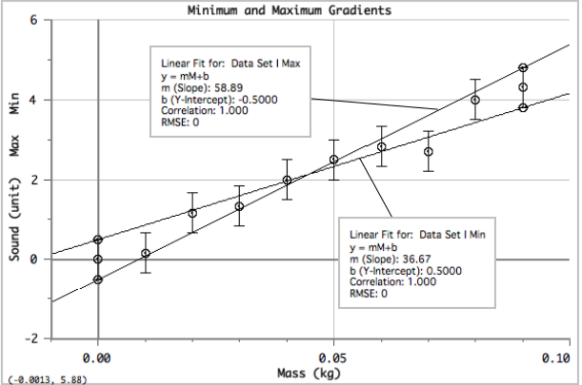13) What is the percent uncertainty if the best fit line slope was 47.44? Round your percentage to the ONES place (DO NOT ADD % SYMBOL) *
14) Stanford University did this lab several times and got a best fit line slope to be 41.33. Calculate the percent error using this number as the accepted value and the number 47.44 as the value from the lab. Round your percentage to the ONES place (DO NOT ADD % SYMBOL) *